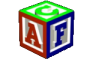About Store Forum Documentation Contact3DRaddictMemberPost: #1 Shader Editor ? I see that a Shader Editor has been on your roadmap for some time now. I would, respectfully, like to suggest that you move it to a higher priority. Through the little experience I've had so far in putting together a custom shader, its a real pain in the arse! The whole idea, surely, is to make it easier for a person whose had at least a small amount of HLSL coding experience. Why should one have to wade backwards and forwards trying to sort through all the EE shader code in its unusually weird format? For instance, providing the simple equivalents of the accepted HLSL global essentials: ModelWorld : world matrix ModelViewMatrix : model view matrix ModelViewProj : view projection matrix ModelViewIT : view inverse transform matrix and texture samplers, for example: sampler HMapSampler = sampler_state { Texture = ; MinFilter = LINEAR; MagFilter = LINEAR; MipFilter = LINEAR; AddressU = WRAP; AddressV = WRAP; }; samplerCUBE sky = sampler_state { Texture = ; MipFilter = NONE; MinFilter = LINEAR; MagFilter = LINEAR; AddressU = WRAP; AddressV = WRAP; AddressW = WRAP; }; sampler fresnel = sampler_state { Texture = ; MipFilter = NONE; MinFilter = LINEAR; MagFilter = LINEAR; AddressU = CLAMP; AddressV = CLAMP; }; sampler reflmap = sampler_state { Texture = ; MipFilter = LINEAR; MinFilter = LINEAR; MagFilter = LINEAR; AddressU = CLAMP; AddressV = CLAMP; }; sampler refrmap = sampler_state { Texture = ; MipFilter = LINEAR; MinFilter = LINEAR; MagFilter = LINEAR; AddressU = CLAMP; AddressV = CLAMP; }; And the means to access them, for example: Vec4 color = tex2D(HMapSampler,vtx.tex()) Vec4 reflColor = texCUBE(EnvMapSampler,Vec4(reflVect.x, reflVect.y, reflVect.z, 1.0))
09-15-2014 06:41 PM
Esenthel3DRaddict